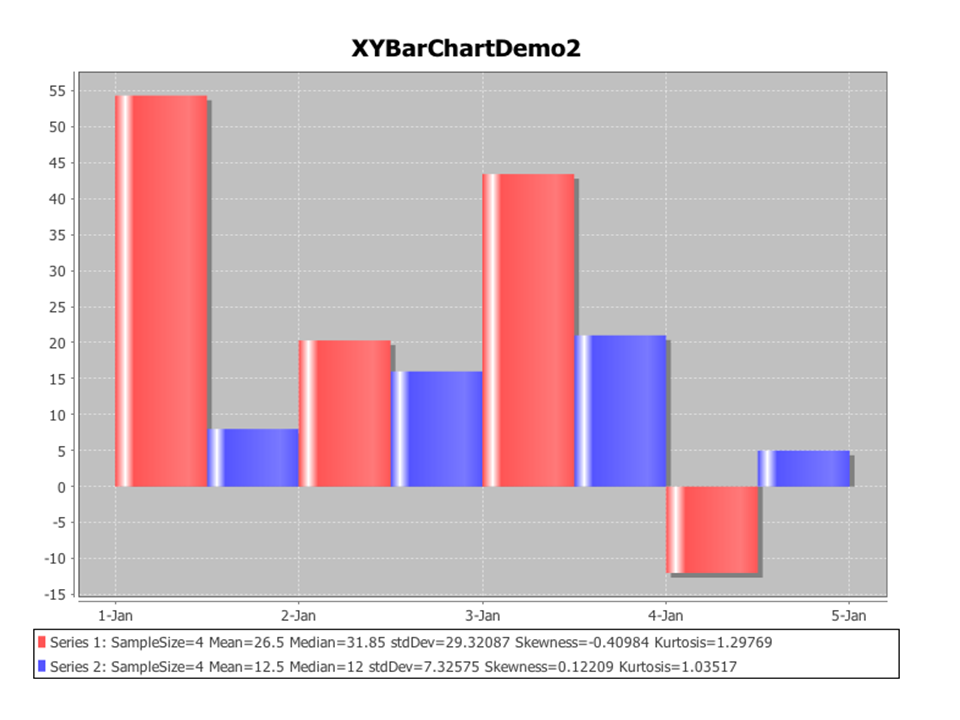# Difference between revisions of "SMHS EDA"

## Scientific Methods for Health Sciences - Exploratory Data Analysis (EDA), Charts and Plots

### Overview

• What is data? Data is a collection of facts, observations or information, such as values or measurements. Data can be numbers, measurements, or even just description of things (meta-data). Data types can be divided into two big categories of quantitative (numerical information) and qualitative data (descriptive information).
• Quantitative data is anything that can be expressed as a number, or quantified. For example, the scores on a math test or weight of girls in the fourth grade are both quantitative data. Quantitative data (discrete or continuous) is often referred to as the measurable data and this type of data allows scientists to perform various arithmetic operations, such as addition, multiplication, functional-evaluation, or to find parameters of a population. There are two major types of quantitative data: discrete and continuous.
• Discrete data results from either a finite, or infinite but countable, possible options for the values present in a given discrete data set and the values of this data type can constitute a sequence of isolated or separated points on the real number line.
• Continuous quantitative data results from infinite and dense possible values that the observations can take on.
• Qualitative data cannot be expressed as a number. Examples may be gender, religious preference. Categorical data (qualitative or nominal) results from placing individuals into groups or categories. Ordinal and qualitative categorical data types both fall into this category.

In statistics, exploratory data analysis (EDA) is an approach to analyze data sets to summarize their main characteristics. Modern statistics regards the graphical visualization and interrogation of data as a critical component of any reliable method for statistical modeling, analysis and interpretation of data. Formally, there are two types of data analysis that should be employed in concert on the same set of data to make a valid and robust inference: graphical techniques and quantitative techniques. We will discuss many of these later, but below is a snapshot of EDA approaches:

• Box plot, Histogram; Multi-vari chart; Run chart; Pareto chart; Scatter plot; Stem-and-leaf plot;
• Parallel coordinates; Odds ratio; Multidimensional scaling; Targeted projection pursuit; Principal component analysis; Multi-linear PCA; Projection methods such as grand tour, guided tour and manual tour.
• Median polish, Trimean.

### Motivation

```The feel of data comes clearly from the application of various graphical techniques, which serves as a perfect window to human perspective and sense. The primary goal of EDA is to maximize the analyst’s insight into a data set and into the underlying structure of the data set. To get a feel for the data, it is not enough for the analyst to know what is in the data, he or she must also know what is not in the data, and the only way to do that is to draw on our own pattern recognition and comparative abilities in the context of a series of judicious graphical techniques applied to the data.
```

The main objectives of EDA:

• Suggest hypotheses about the causes of observed phenomena;
• Assess (parametric) assumptions on which statistical inference will be based;
• Support the selection of appropriate statistical tools and techniques;
• Provide a basis for further data collection through surveys and experiments.

### Theory

Many EDA techniques have been proposed, validated and adopted for various statistical methodologies. Here is an introduction to some of the frequently used EDA charts and the quantitative techniques.

• 3.1)Box-and-Whisker plot: It is an efficient way for presenting data, especially for comparing multiple groups of data. In the box plot, we can mark-off the five-number summary of a data set (minimum, 25th percentile, median, 75th percentile, maximum). The box contains the 50% of the data. The upper edge of the box represents the 75th percentile, while the lower edge is the 25th percentile. The median is represented by a line drawn in the middle of the box. If the median is not in the middle of the box then the data are skewed. The ends of the lines (whiskers) represent the minimum and maximum values of the data set, unless there are outliers. Outliers are observations below Q_1-1.5(IQR) or above Q_3+1.5(IQR), where Q_1 is the 25th percentile, Q_3 is the 75th percentile, and IQR=Q_3-Q_1 (the interquartile range). The advantage of a box plot is that it provides graphically the location and the spread of the data set, it provides an idea about the skewness of the data set, and can provide a comparison between variables by constructing a side-by-side box plots.
• 3.2) Histogram: It is a graphical visualization of tabulated frequencies or counts of data within equal spaced partition of the range of the data. It shows what proportion of measurements that fall into each of the categories defined by the partition of the data range space.

<left>Comment: Compare the two series from the histogram above, we can easily tell that the pattern of series 2 if more obvious compared to series 1. Our intuition may come from: series 1 has more extreme values across five days, for example, the values for Jan 1st and Jan 3rd are extremely high (almost 55 for Jan 1st) while that for Jan 4th is almost -12. However values for series 2 are all above 0 and fluctuated between 5 and 20.